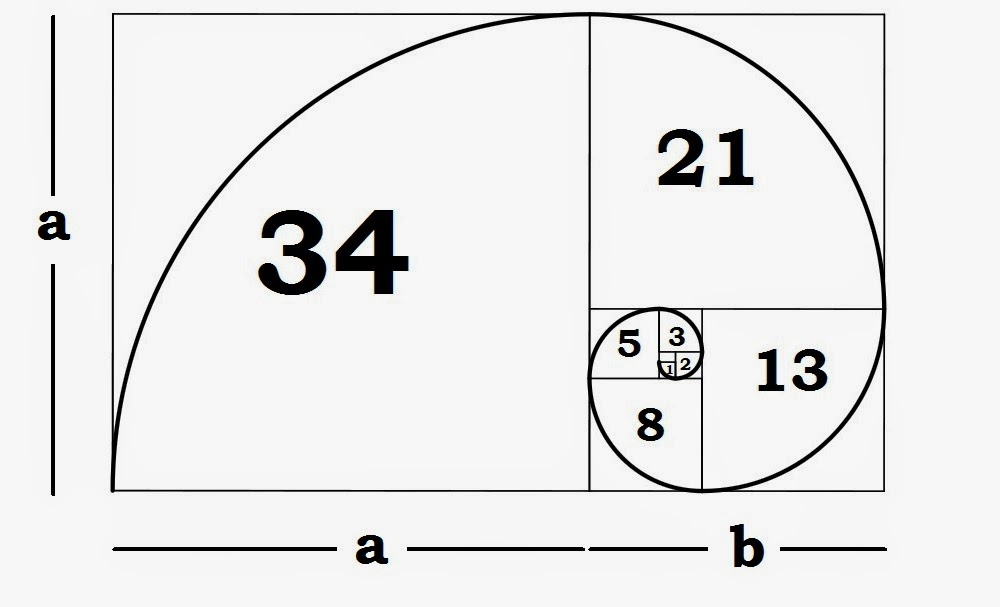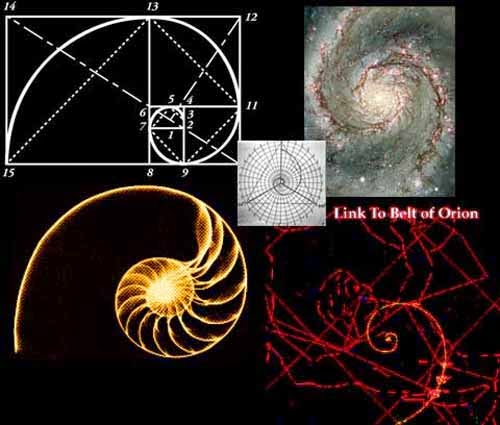Understanding The Fibonacci Sequence & Golden Ratio --># Understanding The Fibonacci Sequence & Golden RatioThe Fibonacci Sequence

The Fibonacci sequence is possibly the most simple recurrence relation occurring in nature. It is 0,1,1,2,3,5,8,13,21,34,55,89, 144… each number equals the sum of the two numbers before it, and the difference of the two numbers succeeding it. It is an infinite sequence which goes on forever as it develops.The Golden Ratio/Divine Ratio or Golden Mean -

The quotient of any Fibonacci number and it's predecessor approaches Phi, represented as ϕ (1.618), the Golden ratio. The Golden Ratio is best understood geometrically by the golden rectangle. A rectangle unevenly divided resulting into one square and one rectangle, the square's sides would have the ratio of 1:1, and the new rectangle would be exactly proportionate to the original rectangle – 1:1.618.

This iteration can continue both ways, infinitely. If you plot a quarter circle inside each of the squares as they reiterate, the golden spiral is formed. The golden spiral is possibly the most simple mathematic pattern that occurs in nature like shells of snails, sea shells, horns, flowers, plants. Numbers are only what we use to organize quantitative information.The Golden Ratio can be applied to any number of geometric forms including circles, triangles, pyramids, prisms, and polygons. The golden ratio is formed by thirds within thirds, sixths, the connection between two and three, including every even and odd number itself. The ratio itself represents the transcendence of numbers, understanding our world is not numbers, but what numbers represent. Through the spiral, the ratio illustrates how the numbers, all quantities, are quality. Eventually, all quality can be represented through quantity. Properties qualitative and quantitative are just labels of information, our gathered indisputable fact.If you graph any number system, eventually patterns appear. In mathematics, numbers and their patterns do not only continue infinitely linear, but in all directions. For example, considering infinite decimal expansion, even the shortest segments have an infinite amount of points.

Our universe and the numbers not only go on infinitely linear, but even it's short segments have infinite points.

The golden ratio is not the only mathematical pattern that reaches infinity, there are many other patterns as well that reach infinity. Knowing this, ask yourself, how could infinity occur twice? If something were to happen infinitely, how could it happen twice? The answer is simple, infinity represents what is eternal, what is truly whole. For example, if infinity were to be used as a variable in mathematics like all other numbers, it would be denoted as 1∞, 2∞, 3∞, 4∞, etc.

The oneness of everything factual is what you know, what you perceive, what you are aware of, is all the universe looking at itself. This is the universe, even you are the universe, us and everything we know is all the same thing.

Since the numbers are everywhere, everything is a part of a pattern. Reflections of reflections, wheels within wheels. Life itself is a Fractal.

(Another video on the Egyptian ‘Temple of Man’ is a symbolic representation of the Fibonacci series of balanced organic expansion and an expression of the underlying principles of life in the universe)

Category:This site's content is licensed under a Creative Commons Attribution License | Terms of Service | Contact Us